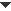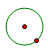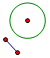﻿ Objects > Circles

# CirclesCircles are fundamental objects in Euclidean geometry. In classical geometric constructions, you use a compass to construct circles. A circle is a path object. You can change the object properties, label properties, and display attributes of circles.Construct a Circle

Sketchpad provides several different ways to construct a circle.

 Use the Compass tool to construct a circle using a center point and another point which defines the radius.Use the Construct | Circle by Center+Point command to construct a circle using a center point and another point which defines the radius.Use the Construct | Circle by Center+Radius command to construct a circle using a center point and either a segment or a distance measurement to define the radius.Use a Circle
 •Construct a point on a circle using the Point tool or the Construct | Point on Object command. Then animate the point around the circle or use the point as the driver for a locus.•Attach an independent point to the circle by using the Edit | Merge command.•Use Construct | Interior to construct the interior of a circle.•Use Construct | Intersection to construct a circle’s intersection with a straight object, another circle, an arc, a point locus, or a function plot.•Use Graph | Define Unit Circle to define a coordinate system that uses the selected circle as its unit circle.Measure a Circle
 Use the Measure menu to find: •the Circumference of a circle•the Radius of a circle•the Area of a circle•the Equation of a circle